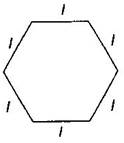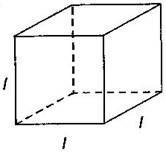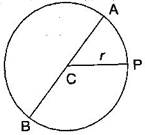### Algebra-Solutions Ex-11.2

CBSE Class –VI Mathematics
NCERT Solutions
Chaper 11 Algebra (Ex. 11.2)

Question 1. The side of an equilateral triangle is shown by $l.$ Express the perimeter of the equilateral triangle using $l.$
Answer: Side of equilateral triangle = $l$
Therefore, Perimeter of equilateral triangle = 3 x side = $3l$
Question 2. The side of a regular hexagon is denoted by $l.$ Express the perimeter of the hexagon using $l.$ (Hint: A regular hexagon has all its six sides in equal length)Answer: Side of hexagon = $l$
Therefore, Perimeter of Hexagon = 6 x side = $6l$
Question 3. A cube is a three-dimensional figure. It has six faces and all of them are identical squares. The length of an edge of the cube is given by $l.$ find the formula for the total length of the edges of a cube.Answer: Length of one edge of cube = $l$
Number of edges in a cube = 12
Therefore, total length = $12×l=12l$
Question 4. The diameter of a circle is a line, which joins two points on the circle and also passes through the centre of the circle. (In the adjoining figure AB is a diameter of the circle; C is its centre). Express the diameter of the circle $\left(d\right)$ in terms of its radius $\left(r\right).$Therefore, $d=2r$ (Here r is the radius of the circle)
This can be done for any three numbers. This property is known as the associativity of addition of numbers. Express this property which we have already studied in the chapter on Whole Numebrs, in a general way, by using variables $a,b$ and $c.$